# Learning to Love Regex

You create a table of information somewhere and decide to transfer it somewhere else in markdown format.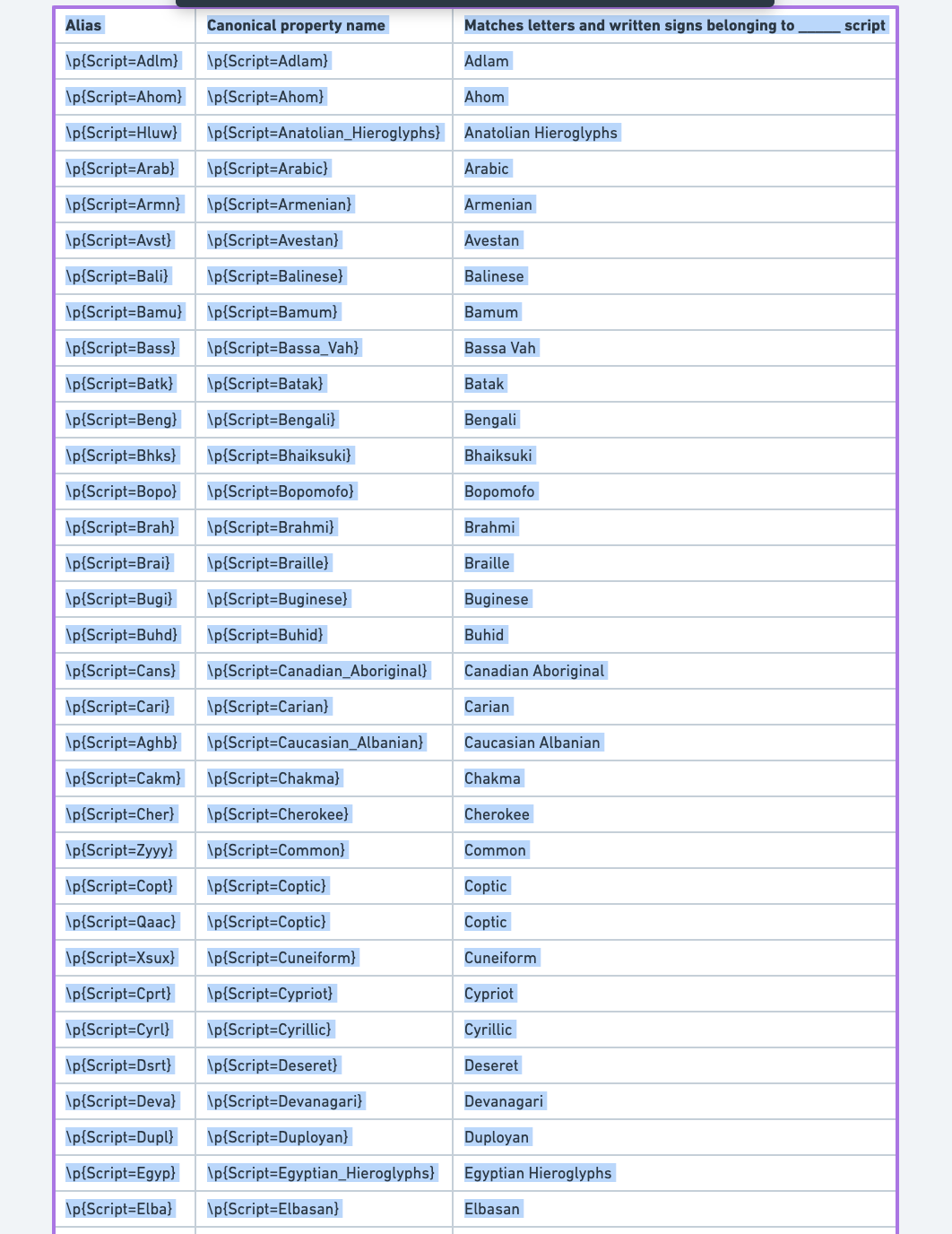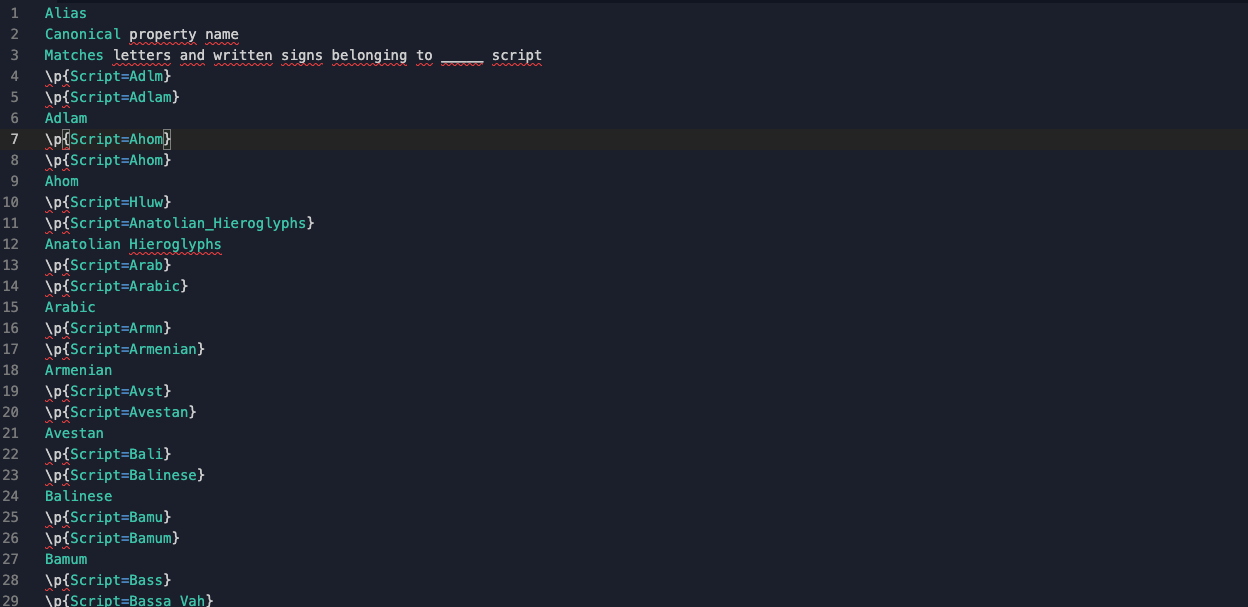Only, when you go to copy the values into your code editor, you realize that the formatting is all wrong! Verdammt! You spent all day compiling this information and you really don't want to spend the rest of the day fiddling around with the formatting to turn it into a markdown table.

Never fear, friend. Regex to the rescue.

## Step 1: Know how to write a markdown table

The basic format is this:

• The heading for the table needs a pipe (|) on either side of each column.
• Between the heading of the table and the table body, there needs to be a line where each column has a pipe on either side and the content of the column has three or more hyphens

Example:

``````| Heading1 | Heading2 |
| --- | --- |
| The most | Basic table ever |
``````

Ends up looking like:

 Heading1 Heading2 The most Basic table ever

## Step 2: Remove newlines

To make subsequent regular expressions easier, remove all the newlines in the pasted text and replace them with a single space.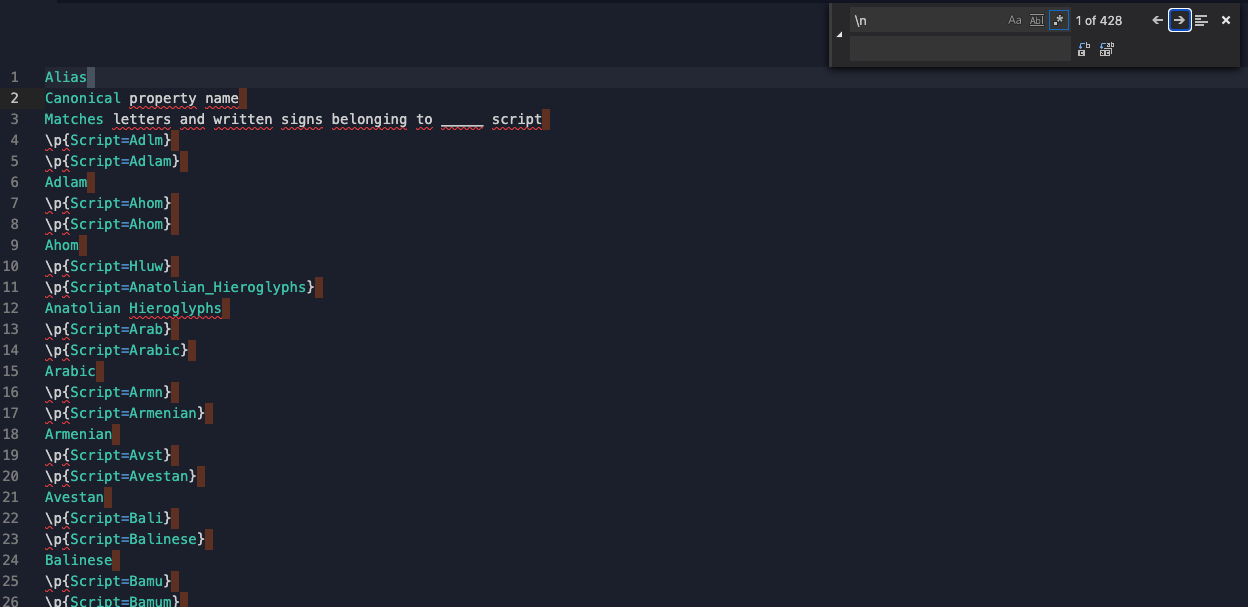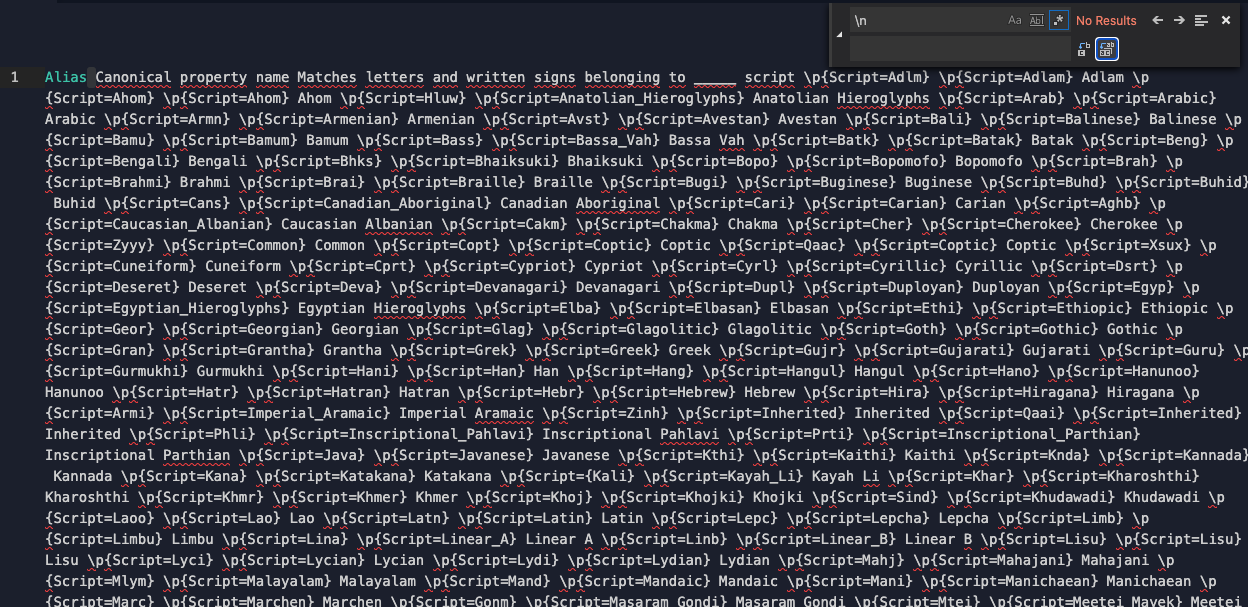## Step 3: Create your table header

This step requires figuring out a regular expression that will match your headers and capture each header individually so that you can manipulate what surrounds it (namely, to add the pipes). This can be accomplished by making use of capture groups. For capture groups, each capture is given a number internally which can then be used in the replace operation. In this example, our table header should include `Alias`, `Canonical property name`, and `Matches letters and written signs belonging to ____ script` as headers, so we need to come up with a way to match those. Note that with regular expressions, there are a ton of ways to approach a regular expression to match text, so this is by no means the only way to go about it.

``````Find:
(\w+) ([\w\s]+(?= Matches)) ([\w\s]+)

Replace:
| \$1 | \$2 | \$3 |\n| --- | --- | --- |\n
``````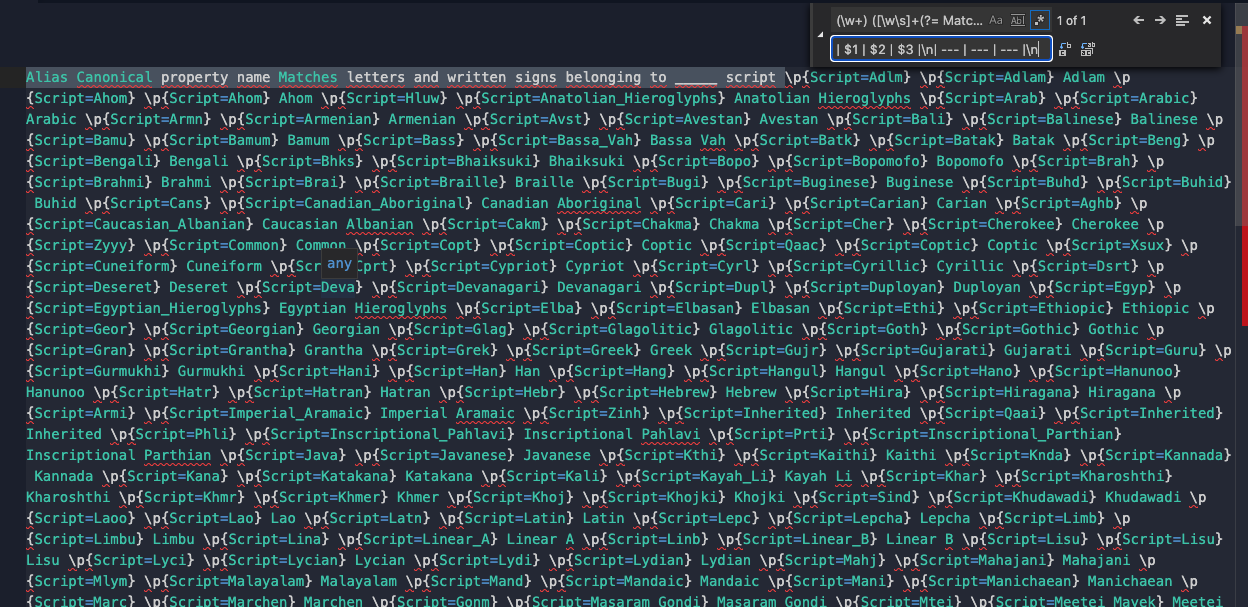The find regex:

• Creates a capture group of one or more alphanumeric characters (including underscore)
• Matches a space
• Creates a second capture group of one or more of either alphanumeric characters (including underscore) or whitespace characters only if it is followed by a space and the word 'Matches' (Since the third heading begins with 'Matches', this is a way to ensure that the second capture group ends at the right spot)
• Matches a space
• Creates a third capture group of one or more either alphanumeric characters (including underscore) or whitespace characters

`Alias` becomes capture group 1 `Canonical property name` becomes capture group 2 `Matches letters and written signs belonging to ____ script` becomes capture group 3

The replacement regex:

• Adds a pipe (|) and a space before capture group 1
• Adds a space and a pipe and a space before capture group 2
• Adds a space and a pipe and a space before capture group 3
• Adds a space and a pipe after the third capture group
• Adds a new line
• Adds a pipe
• Adds a space
• Adds three hyphens
• Adds a space
• Adds a pipe
• Adds a space
• Adds three hyphens
• Adds a space
• Adds a pipe
• Adds a space
• Adds three hyphens
• Adds a space
• Adds a pipe
• Adds a new line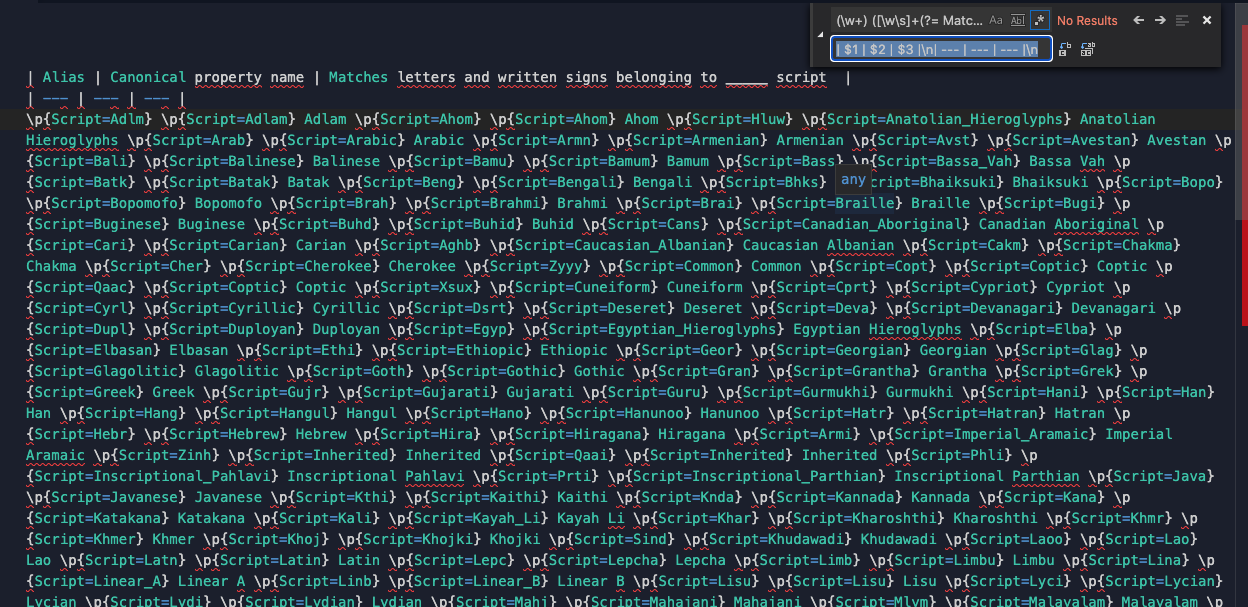## Step 4: Create the table body

This is much like the routine we went through to create the header for the table -- we need to come up with a regular expression that will match what we want to match and ensure that our replacement regular expression converts it into the format we're looking for.

I know from having input all of this data that the pattern for the table is:

• The first column starts with `\p{Script=`, is followed by variable number of letters, followed by `}`
• The second column starts with `\p{Script=`, is followed by a variable number of letters (and/or underscores), followed by `}`
• The third column is a variable number of letters and can include multiple words (so can include whitespace)
``````Find:
(\\p{Script=\w+}) (\\p{Script=\w+}) ([\w\s]+)

Replace:
| \$1 | \$2 | \$3 |\n
``````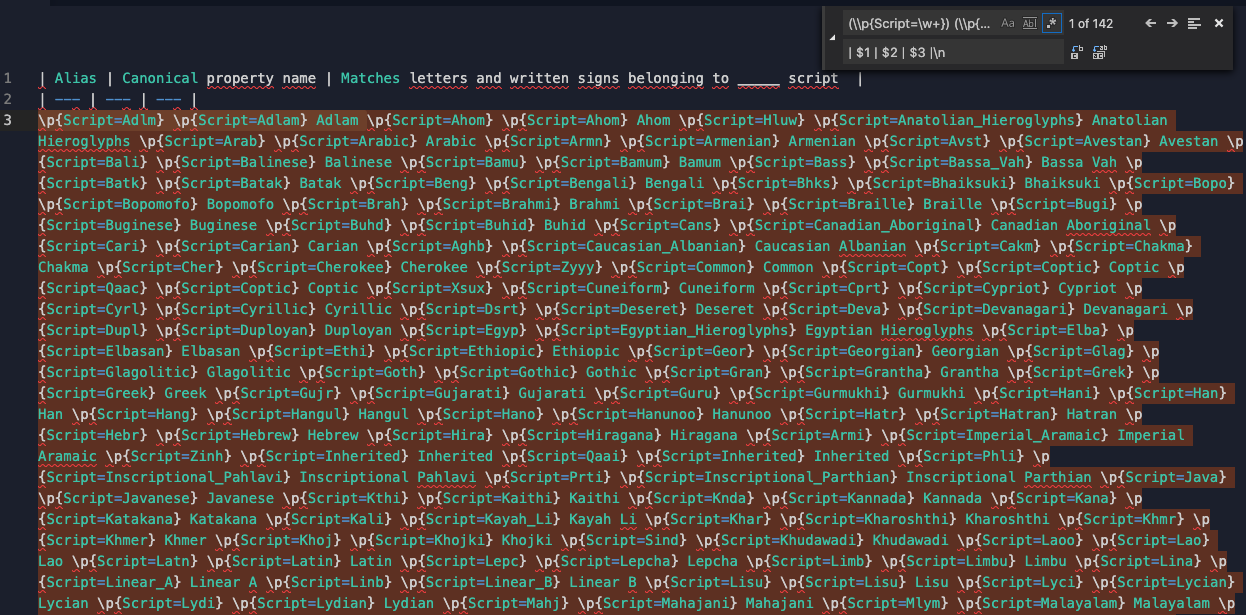The find regex:

• Creates a capture group of the value `\p{Script=` followed by one ore more alphanumeric characters followed by a `}`
• Matches a space
• Creates a capture group of the value `\p{Script=` followed by one or more alphanumeric values followed by a `}`
• Matches a space
• Creates a capture group of one or more alphanumeric values or whitespaces

For the first row of the table: `\p{Script=Adlm}` becomes capture group 1 `\p{Script=Adlam}` becomes capture group 2 `Adlam` becomes capture group 3

The replacement regex:

• Adds a pipe and a space before capture group 1
• Adds a pipe and a space before capture group 2
• Adds a pipe and a space before capture group 3
• Adds a space and a pipe after capture group 3
• Adds a new line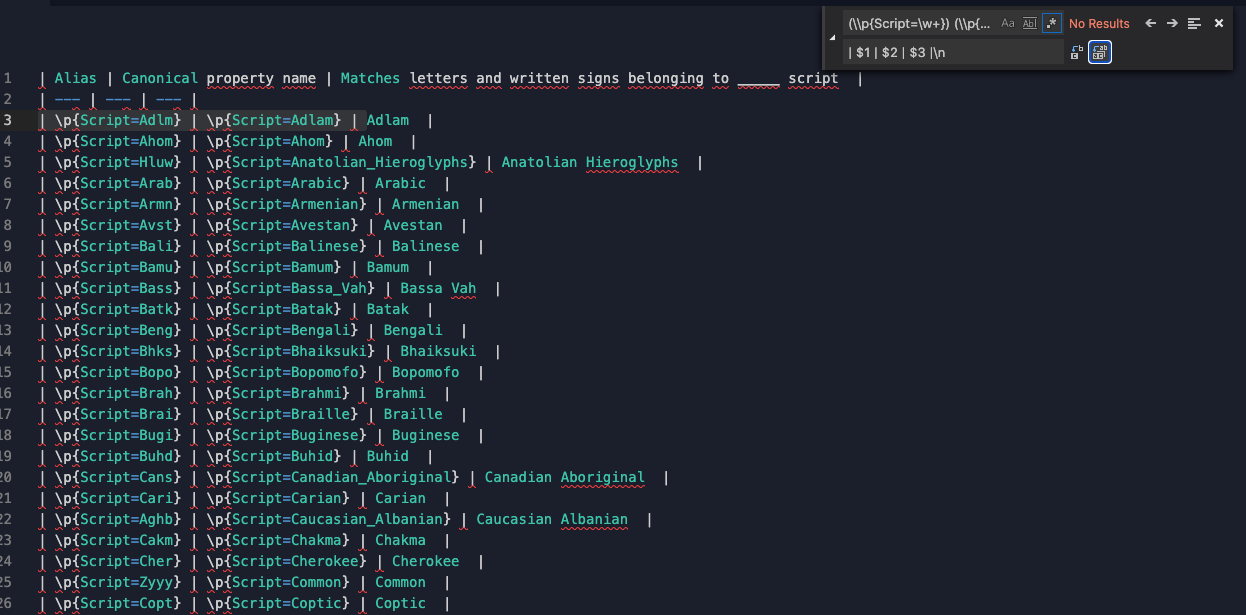Copying that newly formatted text here results in (moment of truth....)

 Alias Canonical property name Matches letters and written signs belonging to _ script \p{Script=Adlm} \p{Script=Adlam} Adlam \p{Script=Ahom} \p{Script=Ahom} Ahom \p{Script=Hluw} \p{Script=Anatolian_Hieroglyphs} Anatolian Hieroglyphs \p{Script=Arab} \p{Script=Arabic} Arabic \p{Script=Armn} \p{Script=Armenian} Armenian \p{Script=Avst} \p{Script=Avestan} Avestan \p{Script=Bali} \p{Script=Balinese} Balinese \p{Script=Bamu} \p{Script=Bamum} Bamum \p{Script=Bass} \p{Script=Bassa_Vah} Bassa Vah \p{Script=Batk} \p{Script=Batak} Batak \p{Script=Beng} \p{Script=Bengali} Bengali \p{Script=Bhks} \p{Script=Bhaiksuki} Bhaiksuki \p{Script=Bopo} \p{Script=Bopomofo} Bopomofo \p{Script=Brah} \p{Script=Brahmi} Brahmi \p{Script=Brai} \p{Script=Braille} Braille \p{Script=Bugi} \p{Script=Buginese} Buginese \p{Script=Buhd} \p{Script=Buhid} Buhid \p{Script=Cans} \p{Script=Canadian_Aboriginal} Canadian Aboriginal \p{Script=Cari} \p{Script=Carian} Carian \p{Script=Aghb} \p{Script=Caucasian_Albanian} Caucasian Albanian \p{Script=Cakm} \p{Script=Chakma} Chakma \p{Script=Cher} \p{Script=Cherokee} Cherokee \p{Script=Zyyy} \p{Script=Common} Common \p{Script=Copt} \p{Script=Coptic} Coptic \p{Script=Qaac} \p{Script=Coptic} Coptic \p{Script=Xsux} \p{Script=Cuneiform} Cuneiform \p{Script=Cprt} \p{Script=Cypriot} Cypriot \p{Script=Cyrl} \p{Script=Cyrillic} Cyrillic \p{Script=Dsrt} \p{Script=Deseret} Deseret \p{Script=Deva} \p{Script=Devanagari} Devanagari \p{Script=Dupl} \p{Script=Duployan} Duployan \p{Script=Egyp} \p{Script=Egyptian_Hieroglyphs} Egyptian Hieroglyphs \p{Script=Elba} \p{Script=Elbasan} Elbasan \p{Script=Ethi} \p{Script=Ethiopic} Ethiopic \p{Script=Geor} \p{Script=Georgian} Georgian \p{Script=Glag} \p{Script=Glagolitic} Glagolitic \p{Script=Goth} \p{Script=Gothic} Gothic \p{Script=Gran} \p{Script=Grantha} Grantha \p{Script=Grek} \p{Script=Greek} Greek \p{Script=Gujr} \p{Script=Gujarati} Gujarati \p{Script=Guru} \p{Script=Gurmukhi} Gurmukhi \p{Script=Hani} \p{Script=Han} Han \p{Script=Hang} \p{Script=Hangul} Hangul \p{Script=Hano} \p{Script=Hanunoo} Hanunoo \p{Script=Hatr} \p{Script=Hatran} Hatran \p{Script=Hebr} \p{Script=Hebrew} Hebrew \p{Script=Hira} \p{Script=Hiragana} Hiragana \p{Script=Armi} \p{Script=Imperial_Aramaic} Imperial Aramaic \p{Script=Zinh} \p{Script=Inherited} Inherited \p{Script=Qaai} \p{Script=Inherited} Inherited \p{Script=Phli} \p{Script=Inscriptional_Pahlavi} Inscriptional Pahlavi \p{Script=Prti} \p{Script=Inscriptional_Parthian} Inscriptional Parthian \p{Script=Java} \p{Script=Javanese} Javanese \p{Script=Kthi} \p{Script=Kaithi} Kaithi \p{Script=Knda} \p{Script=Kannada} Kannada \p{Script=Kana} \p{Script=Katakana} Katakana \p{Script=Kali} \p{Script=Kayah_Li} Kayah Li \p{Script=Khar} \p{Script=Kharoshthi} Kharoshthi \p{Script=Khmr} \p{Script=Khmer} Khmer \p{Script=Khoj} \p{Script=Khojki} Khojki \p{Script=Sind} \p{Script=Khudawadi} Khudawadi \p{Script=Laoo} \p{Script=Lao} Lao \p{Script=Latn} \p{Script=Latin} Latin \p{Script=Lepc} \p{Script=Lepcha} Lepcha \p{Script=Limb} \p{Script=Limbu} Limbu \p{Script=Lina} \p{Script=Linear_A} Linear A \p{Script=Linb} \p{Script=Linear_B} Linear B \p{Script=Lisu} \p{Script=Lisu} Lisu \p{Script=Lyci} \p{Script=Lycian} Lycian \p{Script=Lydi} \p{Script=Lydian} Lydian \p{Script=Mahj} \p{Script=Mahajani} Mahajani \p{Script=Mlym} \p{Script=Malayalam} Malayalam \p{Script=Mand} \p{Script=Mandaic} Mandaic \p{Script=Mani} \p{Script=Manichaean} Manichaean \p{Script=Marc} \p{Script=Marchen} Marchen \p{Script=Gonm} \p{Script=Masaram_Gondi} Masaram Gondi \p{Script=Mtei} \p{Script=Meetei_Mayek} Meetei Mayek \p{Script=Mend} \p{Script=Mende_Kikakui} Mende Kikakui \p{Script=Merc} \p{Script=Meroitic_Cursive} Meroitic Cursive \p{Script=Mero} \p{Script=Meroitic_Hieroglyphs} Meroitic Hieroglyphs \p{Script=Plrd} \p{Script=Miao} Miao \p{Script=Modi} \p{Script=Modi} Modi \p{Script=Mong} \p{Script=Mongolian} Mongolian \p{Script=Mroo} \p{Script=Mro} Mro \p{Script=Mult} \p{Script=Multani} Multani \p{Script=Mymr} \p{Script=Myanmar} Myanmar \p{Script=Nbat} \p{Script=Nabataean} Nabataean \p{Script=Talu} \p{Script=New_Tai_Lue} New Tai Lue \p{Script=Newa} \p{Script=Newa} Newa \p{Script=Nkoo} \p{Script=Nko} Nko \p{Script=Nshu} \p{Script=Nushu} Nushu \p{Script=Ogam} \p{Script=Ogham} Ogham \p{Script=Olck} \p{Script=Ol_Chiki} Ol Chiki \p{Script=Hung} \p{Script=Old_Hungarian} Old Hungarian \p{Script=Ital} \p{Script=Old_Italic} Old Italic \p{Script=Norb} \p{Script=Old_North_Arabian} Old North Arabian \p{Script=Perm} \p{Script=Old_Permic} Old Permic \p{Script=Xpeo} \p{Script=Old_Persian} Old Persian \p{Script=Sarb} \p{Script=Old_South_Arabian} Old South Arabian \p{Script=Orkh} \p{Script=Old_Turkic} Old Turkic \p{Script=Orya} \p{Script=Oriya} Oriya \p{Script=Osge} \p{Script=Osage} Osage \p{Script=Osma} \p{Script=Osmanya} Osmanya \p{Script=Hmng} \p{Script=Pahawh_Hmong} Pahawh Hmong \p{Script=Palm} \p{Script=Palmyrene} Palmyrene \p{Script=Pauc} \p{Script=Pau_Cin_Hau} Pau Cin Hau \p{Script=Phag} \p{Script=Phags_Pa} Phags Pa \p{Script=Phnx} \p{Script=Phoenician} Phoenician \p{Script=Phlp} \p{Script=Psalter_Pahlavi} Psalter Pahlavi \p{Script=Rjng} \p{Script=Rejang} Rejang \p{Script=Runr} \p{Script=Runic} Runic \p{Script=Samr} \p{Script=Samaritan} Samaritan \p{Script=Saur} \p{Script=Saurashtra} Saurashtra \p{Script=Shrd} \p{Script=Sharada} Sharada \p{Script=Shaw} \p{Script=Shavian} Shavian \p{Script=Sidd} \p{Script=Siddham} Siddham \p{Script=Sgnw} \p{Script=SignWriting} SignWriting \p{Script=Sinh} \p{Script=Sinhala} Sinhala \p{Script=Sora} \p{Script=Sora_Sompeng} Sora Sompeng \p{Script=Soyo} \p{Script=Soyombo} Soyombo \p{Script=Sund} \p{Script=Sundanese} Sundanese \p{Script=Sylo} \p{Script=Syloti_Nagri} Syloti Nagri \p{Script=Syrc} \p{Script=Syriac} Syriac \p{Script=Tglg} \p{Script=Tagalog} Tagalog \p{Script=Tagb} \p{Script=Tagbanwa} Tagbanwa \p{Script=Tale} \p{Script=Tai_Le} Tai Le \p{Script=Lana} \p{Script=Tai_Tham} Thai Tham \p{Script=Tavt} \p{Script=Tai_Viet} Tia Viet \p{Script=Takr} \p{Script=Takri} Takri \p{Script=Taml} \p{Script=Tamil} Tamil \p{Script=Tang} \p{Script=Tangut} Tangut \p{Script=Telu} \p{Script=Telugu} Telugu \p{Script=Thaa} \p{Script=Thaana} Thaana \p{Script=Thai} \p{Script=Thai} Thai \p{Script=Tibt} \p{Script=Tibetan} Tibetan \p{Script=Tfng} \p{Script=Tifinagh} Tifinagh \p{Script=Tirh} \p{Script=Tirhuta} Tirhuta \p{Script=Ugar} \p{Script=Ugaritic} Ugaritic \p{Script=Vaii} \p{Script=Vai} Vai \p{Script=Wara} \p{Script=Warang_Citi} Warang Citi \p{Script=Yiii} \p{Script=Yi} Yi \p{Script=Zanb} \p{Script=Zanzabar_Square} Zanzabar Square

So if you find yourself in a situation where you need to format data and you really don't want to manually go through the repetitive work involved.... look for patterns, embrace the regex, and save yourself some time.